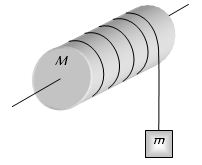# Translation Converted To RotationIn the figure above, a body of mass $m$ is tied at one end of a light string and this string is wrapped around the solid cylinder of mass $M$ and radius $R$. At the moment $t=0$ the system starts moving. If the friction is negligible, then what is the angular velocity at time $t?$

×